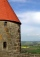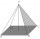# Surface area - high school - math problems

#### Number of problems found: 176

• Sphere in coneA sphere is inscribed in the cone (the intersection of their boundaries consists of a circle and one point). The ratio of the surface of the ball and the contents of the base is 4: 3. A plane passing through the axis of a cone cuts the cone in an isoscele
• The cylinderThe cylinder has a surface area of 300 square meters, while the cylinder's height is 12 m. Calculate the volume of this cylinder.
• The tentCalculate how much cover (without a floor) is used to make a tent that has the shape of a regular square pyramid. The edge of the base is 3 m long and the height of the tent is 2 m.
• Magnified cubeIf the lengths of the cube's edges are extended by 5 cm, its volume will increase by 485 cm3. Determine the surface of both the original and the magnified cube.
• Cone roofHow many m2 of roofing is needed to cover a cone-shaped roof with a diameter of 10 m and a height of 4 m? Add an extra 4% to the overlays.
• Triangular prismThe base of the perpendicular triangular prism is a rectangular triangle with a hypotenuse of 10 cm and one leg of 8 cm. The prism height is 75% of the perimeter of the base. Calculate the volume and surface of the prism.
• Block or cuboidThe wall diagonals of the block have sizes of √29cm, √34cm, √13cm. Calculate the surface and volume of the block.Calculate the volume (V) and the surface (S) of a regular quadrilateral prism whose height is 28.6 cm and the deviation of the body diagonal from the base plane is 50°.
• Triangular prism,The regular triangular prism, whose edges are identical, has a surface of 2514 cm ^ 2 (square). Find the volume of this body in cm3 (l).
• Three faces of a cuboidThe diagonal of three faces of a cuboid are 13,√281, and 20 units. Then the total surface area of the cuboid is.
• Top of the towerThe top of the tower has the shape of a regular hexagonal pyramid. The base edge has a length of 1.2 m, the pyramid height is 1.6 m. How many square meters of sheet metal is needed to cover the top of the tower if 15% extra sheet metal is needed for joint
• The pyramid 4sThe pyramid with a rectangular base measuring 6 dm and 8 dm has a side edge of length 13 dm. Calculate the surface area and volume of this pyramid.
• Storm and roofThe roof on the building is a cone with a height of 3 meters and a radius equal to half the height of the roof. How many m2 of roof need to be repaired if 20% were damaged in a storm?
• The bus stopThe bus stop waiting room has the shape of a regular quadrilateral pyramid 4 m high with a 5 m base edge. Calculate how much m2 roofing is required to cover the sheathing three walls, taking 40% of the additional coverage.
• The ballThe ball has a radius of 2m. What percentage of the surface and volume is another sphere whose radius is 20% larger?
• Axial section of the coneThe axial section of the cone is an isosceles triangle in which the ratio of cone diameter to cone side is 2: 3. Calculate its volume if you know its area is 314 cm square.
• Cone sideCalculate the volume and area of the cone whose height is 10 cm and the axial section of the cone has an angle of 30 degrees between height and the cone side.
• PoolHow many hl of water is in a cuboid pool (a = 25m, b = 8m) if the area of the wetted walls is 279.2 m2?
• Tetrahedral pyramidDetermine the surface of a regular tetrahedral pyramid when its volume is V = 120 and the angle of the sidewall with the base plane is α = 42° 30'.
• Surface of the cylinderCalculate the surface of the cylinder for which the shell area is Spl = 20 cm2 and the height v = 3.5 cm

Do you have an interesting mathematical word problem that you can't solve? Submit a math problem, and we can try to solve it.

We will send a solution to your e-mail address. Solved examples are also published here. Please enter the e-mail correctly and check whether you don't have a full mailbox.

Please do not submit problems from current active competitions such as Mathematical Olympiad, correspondence seminars etc...

Examples for the calculation of the surface area of ​​the solid object . Examples for secondary school students.